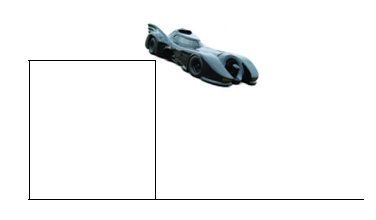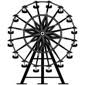# Pre-ap physics 2d motion Homework

 Name: __________________________ Period: _____ Pre-AP Physics 2D Motion Homework Instructions: Answer each question in the space provided. Be sure to show all work (GUESS) and box your answer(s). This homework assignment is due on 10/1/2010. Part I: Projectile Motion Batman drives the Bat-mobile off the roof of a building in Gotham City at a velocity of 35.2 m/s and hits the street 3.11 seconds later.How tall is the building? How far away from the bottom of the building does he land? A Bomber is practicing a bombing run. The plane is flying with a horizontal velocity of 185 m/s, and releases a bomb when it is 593 m away (horizontally) from its target and scores a direct hit. How high is the airplane flying (dy) when it drops the bomb? A sniper fires a bullet parallel to the ground. If the barrel is 0.25 meters off the ground and the initial velocity is 250 m/s, how far does the bullet travel horizontally before it hits the ground?A rock is kicked from the ground with a velocity of 15 m/s at an angle of 15o above the horizontal. What is the range (dx) of the rock? Tiger Woods is at it again! He hits a golf ball with a velocity of 25 m/s at an angle of 65o. What is the range of Tiger’s golf ball?A trebuchet fires a projectile at an angle of 50o with a velocity of 68 m/s. What is the maximum height (dy) the projectile reaches at the apex of its trajectory? Part II: Circular Motion A Ferris wheel turns 480o before stopping. How many radians is this?A child on a merry go round completes 1 revolution in 45 seconds. What is the angular velocity of the merry go round? The wheel of a car in motion has an angular velocity of 6.95 rad/s. What is the angular displacement of the wheel after 90 seconds of rotation at this speed? Two coins: a quarter and a penny, are sitting on an old-school record player as it spins an old-school record. The quarter is 0.05 meters from the center and the penny is 0.13 meters from the center. The record player completes one rotation every 8 seconds as it plays.Share with your friends: